# 基于云平台的密文数据离群点检测算法Outlier Detection Algorithm for Cryptographic Data Based on Cloud Platform

DOI: 10.12677/CSA.2021.112032, PDF, HTML, XML, 下载: 55  浏览: 90  国家科技经费支持

Abstract: Outsourcing data and computing to cloud servers for large-scale data storage and query processing is an economical and efficient method. However, due to security and privacy issues, we need to protect sensitive data such as medical records in the process of using cloud server computing. One ap-proach is to outsource the encrypted data to a cloud server and have the cloud server perform only query processing on the encrypted data, so that the cloud server does not gain any knowledge of the data, queries, and query results. The query task of encrypting data without data cloud decryption is very challenging. The task we designed is to detect outliers with the cloud server’s secure k-nearest neighbor (KNN) query method under the condition of encrypted data, that is, the user sends an encrypted query to the cloud server with encrypted data set and obtains the information whether the data is an outlier or not. We first propose a simple scheme and prove that the scheme is not completely safe. Then we proposed a security scheme with higher security, which can effectively protect the confidentiality of user query data, server data set and data access mode.

1. 引言

1) 数据机密性——数据库数据集T或任何中间结果的内容不应向服务器公开。

2) 查询隐私——输入查询数据Q不应该被泄露给服务器。

3) 正确性——输出应该只透露给客户端用户。并且，除了此信息没有其他信息透露给Bob。

4) 用户上的计算开销尽可能低——将加密的查询记录发送到服务器后，我们的协议在客户端上的计算开销比现有的工作  低。

5) 隐藏的数据访问模式——对数据的访问模式，例如查询数据Q的k近邻对应的记录，不应该向数据拥有者和服务器公开(以防止任何推理攻击)。

2. 基础知识

2.1. Paillier加密

paillier加密基于复合剩余类的难解性，是1999年paillier发明的概率公钥加密系统。该加密算法是一种同态加密，满足加法和数乘同态。其同态性质可描述为：

(1) ${E}_{pk}\left(a+b\right)←{E}_{pk}\left(a\right)\ast {E}_{pk}\left(b\right)\mathrm{mod}{N}^{2}$

(2) ${E}_{pk}\left(a\ast b\right)←{E}_{pk}{\left(a\right)}^{b}\mathrm{mod}{N}^{2}$

2.2. 安全乘法(SM)协议

P1包含 ${E}_{pk}\left(a\right)$${E}_{pk}\left(b\right)$，且P1和P2不知道a和b的具体数值，经过P1、P2协同计算后将 ${E}_{pk}\left(a\ast b\right)$ 输出到P1，并且在这个过程中，P1和P2没有得到关于a和b的信息。输出 ${E}_{pk}\left(a\ast b\right)$ 只有P1知道。安全乘法(SM)协议的基本思想基于以下性质，对于任意给定的 $a,b\in {Z}_{N}$，有：

$a\ast b=\left(a+{r}_{a}\right)\ast \left(b+{r}_{b}\right)-a\ast {r}_{b}-b\ast {r}_{a}-{r}_{a}\ast {r}_{b}$

2.3. 安全平方欧几里得距离协议

P1包含 ${E}_{pk}\left(X\right)$${E}_{pk}\left(Y\right)$，其与P2安全地协同计算向量X和Y之间欧氏距离的平方加密。这里X和Y是m维向量，其中：

(1) ${E}_{pk}\left(X\right)=〈{E}_{pk}\left({X}_{1}\right),\cdots ,{E}_{pk}\left({X}_{m}\right)〉$

(2) ${E}_{pk}\left(Y\right)=〈{E}_{pk}\left({Y}_{1}\right),\cdots ,{E}_{pk}\left({Y}_{m}\right)〉$

${|X-Y|}^{2}=\underset{i=1}{\overset{m}{\sum }}{\left({x}_{i}-{y}_{i}\right)}^{2}$

2.4. 安全位分解(SBD)协议

P1包含 ${E}_{pk}\left(z\right)$，其与P2安全地协同计算z的各个比特位的加密，其中 $0\le Z<{2}^{l}$。输出 $\left[z\right]=〈{E}_{pk}\left({z}_{1}\right),\cdots ,{E}_{pk}\left({z}_{l}\right)〉$ 只有P1知道。这里z1和zl分别表示整数z的最高有效位和最低有效位。

2.5. 安全最小(SMIN)协议

P1包含([u]，[v])，服务器 P2包含私钥sk，两个服务器协同安全计算u和v之间小的那一个数的各个比特位的加密，即输出为[min(u,v)]，且该结果只有P1知道。在这个协议中，P1和P2无法得到关于u和v的信息。

2.6. 安全最小n数字(SMINn)协议

P1包含n个加密向量 $\left(\left[{d}_{1}\right],\cdots ,\left[{d}_{n}\right]\right)$，P2包含私钥sk，这里 $\left[{d}_{i}\right]=〈{E}_{pk}\left({d}_{i,1}\right),\cdots ,{E}_{pk}\left({d}_{i,l}\right)〉$，其中 ${d}_{i,1}$${d}_{i,l}$ 分别为整数di的最高和最低有效位，且 $1\le i\le n$。P1和P2协同计算输出 $\left[\mathrm{min}\left({d}_{1},\cdots ,{d}_{n}\right)\right]$。最后， $\left[\mathrm{min}\left({d}_{1},\cdots ,{d}_{n}\right)\right]$ 仅为P1所知晓。并且在SMINn协议过程中，P1和P2无法获取有关di的信息。

2.7. 安全位或(SBOR)协议

P1包含 $\left({E}_{pk}\left({o}_{1}\right),{E}_{pk}\left({o}_{2}\right)\right)$，其与P2安全地协同计算 ${E}_{pk}\left({o}_{1}\vee {o}_{2}\right)$，其中o1和o2是两个二进制数值。输出 ${E}_{pk}\left({o}_{1}\vee {o}_{2}\right)$ 只有P1知道。

3. 方案设计

3.1. 基本安全协议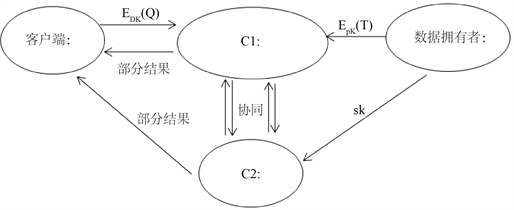Figure 1. Parties setup of the agreement

${E}_{pk}\left(Q\right)=〈{E}_{pk}\left({q}_{1}\right),\cdots ,{E}_{pk}\left({q}_{m}\right)〉$

${E}_{pk}\left({t}_{i}\right)=〈{E}_{pk}\left({t}_{i,1}\right),\cdots ,{E}_{pk}\left({t}_{i,m}\right)〉$

1) 选择加密记录 ${E}_{pk}\left({t}_{{i}_{1}}\right),\cdots ,{E}_{pk}\left({t}_{{i}_{k}}\right)$ 作为查询数据Q的K最近邻记录，并随机化它们的属性。具体而言，C1计算 ${\gamma }_{j,h}={E}_{pk}\left({t}_{{i}_{j},h}\right)\ast {E}_{pk}\left({r}_{j,h}\right)$$1\le j\le k$$1\le h\le m$。这里 ${r}_{j,h}$ 是ZN范围内的一个随机数， ${t}_{{i}_{j},h}$ 表示列h中 ${t}_{{i}_{j}}$ 属性值。然后C1将 ${\gamma }_{j,h}$ 发送到 C2， ${r}_{j,h}$ 发送给Bob，其中 $1\le j\le k$$1\le h\le m$

2) 收到 ${\gamma }_{j,h}$ 之后，服务器C2解密计算 ${{\gamma }^{\prime }}_{j,h}={D}_{sk}\left({\gamma }_{j,h}\right)$，并将其发送给Bob。注意，因为C1之前将 ${\gamma }_{j,h}$ 的加密数值随机化，所以 ${{\gamma }^{\prime }}_{j,h}$ 仍然是ZN范围内的随机数。

3) Bob收到 ${r}_{j,h}$${{\gamma }^{\prime }}_{j,h}$ 之后，使用 ${{t}^{\prime }}_{j,h}={{\gamma }^{\prime }}_{j,h}-{r}_{j,h}\mathrm{mod}N$ 来计算查询数据Q的K近邻数据值，其中 $1\le j\le k$$1\le h\le m$，之后Bob根据得到的K个数值进行离群点的检测，我们采用距离方法顺序进行检测，即从这组数据的第一个开始进行数值欧式距离的判定，一旦存在某一个数值ti与Q之间的欧式距离大于预设的距离D， $1\le i\le k$，则说明在数据群T中与查询数据Q的欧式距离小于D的点不足k个，判定Q为离群点，否则，Q不是离群点。

3.2. 完全安全协议

(1): C1自客户端Bob处接收Epk(Q)

(2): for i=1 to n:

${E}_{pk}\left({d}_{i}\right)=\text{SSED}\left({E}_{pk}\left(Q\right),{E}_{pk}\left({t}_{i}\right)\right)$

$\left[{d}_{i}\right]=〈{E}_{pk}\left({d}_{i,1}\right),\cdots ,{E}_{pk}\left({d}_{i,l}\right)〉=\text{SBD}\left({E}_{pk}\left({d}_{i}\right)\right)$

for s=1 to k do:

(a): C1和C2:

$\left[{d}_{\mathrm{min}}\right]={\text{SMIN}}_{\text{n}}\left(\left[{d}_{1}\right],\cdots ,\left[{d}_{n}\right]\right)$

(b): C1:

${E}_{pk}\left({d}_{\mathrm{min}}\right)={\prod }_{\gamma =0}^{l-1}{E}_{pk}{\left({d}_{\mathrm{min},\gamma +1}\right)}^{{2}^{l-\gamma -1}}$

${E}_{pk}\left({d}_{i}\right)={\prod }_{\gamma =0}^{l-1}{E}_{pk}{\left({d}_{i,\gamma +1}\right)}^{{2}^{l-\gamma -1}},s\ne 1,1\le i\le n$

for i=1 to n:

${\tau }_{i}={E}_{pk}\left({d}_{\mathrm{min}}\right)\ast {E}_{pk}{\left({d}_{i}\right)}^{N-1}$

${{\tau }^{\prime }}_{i}={\tau }_{i}^{{r}_{i}}={E}_{pk}\left({r}_{i}\ast \left({d}_{\mathrm{min}}-{d}_{i}\right)\right)$

$\beta =\pi \left({\tau }^{\prime }\right)$，将β发送至C2

(c): C2:

${{\beta }^{\prime }}_{i}={D}_{sk}\left({\beta }_{i}\right),1\le i\le n$

for $1\le i\le n$ :

if ${{\beta }^{\prime }}_{i}=0$ : ${U}_{i}={E}_{pk}\left( 1 \right)$

else ${U}_{i}={E}_{pk}\left( 0 \right)$

(d): C1:

${{V}^{\prime }}_{i,j}=\text{SM}\left({V}_{i},{E}_{pk}\left({t}_{i,j}\right)\right),1\le i\le n,1\le j\le m$

${E}_{pk}\left({{t}^{\prime }}_{i,j}\right)={\prod }_{i=1}^{n}{{V}^{\prime }}_{i,j},1\le j\le m$

${E}_{pk}\left({{t}^{\prime }}_{s}\right)=〈{E}_{pk}\left({{t}^{\prime }}_{s,1}\right),\cdots ,{E}_{pk}\left({{t}^{\prime }}_{s,m}\right)〉$

(e): C1和C2:

${E}_{pk}\left({d}_{i,\gamma }\right)=\text{SBOR}\left({V}_{i},{E}_{pk}\left({d}_{i,\gamma }\right)\right),1\le i\le n,1\le \gamma \le l$ 剩余算法步骤与SkNNb的4~6步相同

1) 使用下面的公式计算加密数值dmin

${E}_{pk}\left({d}_{\mathrm{min}}\right)=\underset{\gamma =0}{\overset{l-1}{\prod }}{E}_{pk}{\left({d}_{\mathrm{min},\gamma +1}\right)}^{{2}^{l-\gamma -1}}={E}_{pk}\left({d}_{\mathrm{min},1}\ast {2}^{l-1}+\cdots +{d}_{\mathrm{min},l}\right)$

2) 计算加密数据dmin和每一个距离数据di的差别，即在 $1\le i\le n$ 范围内计算

${\tau }_{i}={E}_{pk}\left({d}_{\mathrm{min}}\right)\ast {E}_{pk}{\left({d}_{i}\right)}^{N-1}={E}_{pk}\left({d}_{\mathrm{min}}-{d}_{i}\right)$

3) 将 ${\tau }_{i}$ 随机化，具体表示为 ${{\tau }^{\prime }}_{i}={\tau }_{i}^{{r}_{i}}={E}_{pk}\left({r}_{i}\ast \left({d}_{\mathrm{min}}-{d}_{i}\right)\right)$，ri为ZN范围内的随机数。注意， ${{\tau }^{\prime }}_{i}$ 是一个加密过的0或一个随机数，其中 $1\le i\le n$。同时，使用随机置换函数π(该函数只有C1知道)将其乱序，得到 $\beta =\pi \left({\tau }^{\prime }\right)$ 并发送到C2。

1) 服务器C1和C2共同参与安全乘法(SM)协议，计算 ${{V}^{\prime }}_{i,j}={V}_{i}\ast {E}_{pk}\left({t}_{i,j}\right)$，范围为 $1\le i\le n$$1\le j\le m$。安全乘法SM协议的输出结果 ${V}^{\prime }$ 只有C1知道。然后，服务器C1通过使用paillier加密的同态属性，计算加密数据记录 ${E}_{pk}\left({{t}^{\prime }}_{1}\right)=〈{E}_{pk}\left({t}_{1,1}\right),\cdots ,{E}_{pk}\left({t}_{1,m}\right)〉$，其中 ${E}_{pk}\left({{t}^{\prime }}_{i,j}\right)={\prod }_{i=1}^{n}{{V}^{\prime }}_{i,j}$，其中 $1\le j\le m$${{t}^{\prime }}_{1,j}$${{t}^{\prime }}_{1}$ 的第j个属性值。

2) 我们需要注意，最接近查询数据Q的那一个元组应该被排除在后续的计算之外。但是，由于服务器C1不知道与 ${E}_{pk}\left({{t}^{\prime }}_{1}\right)$ 对应的数据记录，因此我们需要强制消除在下一次迭代运算中再次选择该数据记录的可能性。对此，我们让C1将 ${E}_{pk}\left({{t}^{\prime }}_{1}\right)$ 对应的距离更新为最大值，即2l − 1。更具体地说，C1在C2的帮助下，使用安全位或SBOR协议在范围 $1\le i\le n$$1\le \gamma \le l$ 内更新距离向量 ${E}_{pk}\left({d}_{i,\gamma }\right)=\text{SBOR}\left({V}_{i},{E}_{pk}\left({d}_{i,\gamma }\right)\right)$。当 ${V}_{i}={E}_{pk}\left(\text{1}\right)$ 时，相应的距离向量di设置为最大值。也就是说，在这种情况下， $\left[{d}_{i}\right]=〈{E}_{pk}\left(1\right),\cdots ,{E}_{pk}\left(1\right)〉$。但是，当 ${V}_{i}={E}_{pk}\left(0\right)$ 时，OR操作对di没有影响。

Bob收到 ${r}_{j,h}$${{\gamma }^{\prime }}_{j,h}$ 之后，使用 ${{t}^{\prime }}_{j,h}={{\gamma }^{\prime }}_{j,h}-{r}_{j,h}\mathrm{mod}N$ 来计算查询数据Q的K近邻数据值，其中 $1\le j\le k$$1\le h\le m$，之后Bob根据得到的K个数值进行离群点的检测，我们采用距离方法顺序进行检测，即从这组数据的第一个开始进行数值欧式距离的判定，一旦存在某一个数值ti与Q之间的欧式距离大于预设的距离D， $1\le i\le k$，则说明在数据群T中与查询数据Q的欧式距离小于D的点不足k个，判定Q为离群点，否则，Q不是离群点。

4. 实验分析

4.1. 安全性分析

4.2. 复杂性分析

SkNNb的计算复杂度受加密、解密和求幂的限制为 $O\left(n\ast m+k\right)$。实际上 $k\ll n\ast m$，因此，受到加密和求幂的限制(假设在Pallier密码系统下加密和解密操作需要相同的时间)SkNNb的计算复杂度为 $O\left(n\ast m\right)$。另一方面，SkNNm的计算复杂度受加密和取幂的限制为 $O\left(n\ast \left(l+m+k\ast l\ast {\mathrm{log}}_{2}n\right)\right)$

4.3. 数据对比

1) SkNNb协议测试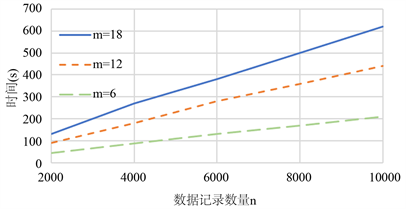Figure 2. SkNNb trend chart in Case k = 5, S = 512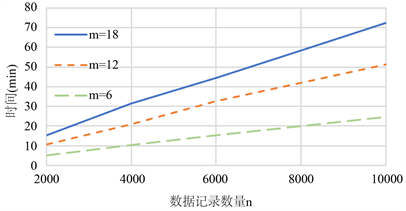Figure 3. SkNNb Trend Chart in Case k = 5, S = 1024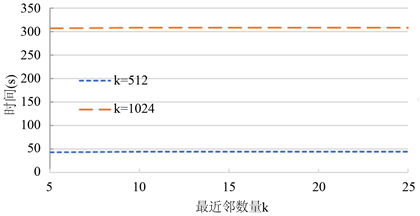Figure 4. SkNNb Trend Chart in Case m = 6, n = 2000

2) SkNNm协议测试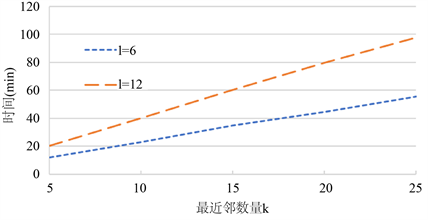Figure 5. SkNNm trend chart in Case n = 2000, S = 512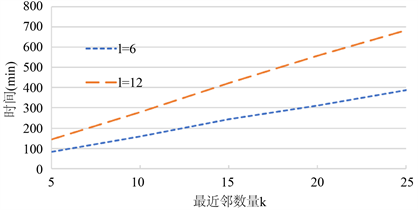Figure 6. SkNNm trend chart in Case n = 2000, S = 1024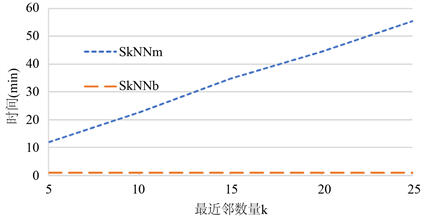Figure 7. Trend comparison chart between SkNNm and SkNNb

5. 总结

k近邻是离群点检测常用的查询方法之一。在将加密数据存储在数据库环境中，对加密数据的安全查询处理变得很有挑战性。现有的基于加密数据的SkNN技术是不安全的。在本文中，我们使用了两种新的基于加密数据的SkNN协议进行离群点的检测。作为基本解决方案的第一种协议向服务器泄漏了一些信息。但是，我们使用的第二个协议是完全安全的，能够保护数据、用户输入查询的机密性，并隐藏数据访问模式。然而，第二种协议比基本协议计算成本更高。此外，我们还评估了协议在不同参数设置下的性能。

  Oppliger, R. (2003) Microsoft.net Passport: A Security Analysis. Computer, 7, 29-35. https://doi.org/10.1109/MC.2003.1212687  Ellin, B. (2006) About openlD. http://www.openidenabled.com/openid/about-openid  ShoCardBlockchain Identity Management White Paper. https://shocard.com/blockchain-identity-whitepapers/  Erlingsson, U., Pihur, V. and Korolova, A. (2014) Rap-por: Randomized Aggregatable Privacy-Preserving Ordinal Response. ACM SIGSAC Conference on Computer and Communications Security, 1054-1067. https://doi.org/10.1145/2660267.2660348  Warner, S.L. (1965) Randomized Response: A Survey Technique for Eliminating Evasive Answer Bias. Journal of the American Statistical Association, 60, 63-66. https://doi.org/10.1080/01621459.1965.10480775  Dwork, C. (2006) Differential Privacy. International Con-ference on Automata, Languages and Programming, 1-12. https://doi.org/10.1007/11787006_1  Dwork, C., McSherry, F., Nissim, K. and Smith, A. (2006) Calibrating Noise to Sensitivity in Private Data Analysis. Theory of Cryptography Conference, 265-284. https://doi.org/10.1007/11681878_14  Duchi, J.C., Jordan, M.I. and Wainwright, M.J. (2013) Local Privacy and Statistical Minimax Rates. IEEE FOCS, 429- 438. https://doi.org/10.1109/FOCS.2013.53  Liu, J.F., Yang, J.C. and Li, X. (2018) Secure and Efficient Skyline Queries on Encrypted Data, TKDE.